﻿ Linear Mix-net and a Scheme for Collecting Data from Anonymous Data Holders

### Linear Mix-net and a Scheme for Collecting Data from Anonymous Data Holders

Shinsuke Tamura, Shuji TaniguchiOPEN ACCESSPEER-REVIEWED

## Linear Mix-net and a Scheme for Collecting Data from Anonymous Data Holders

Shinsuke Tamura1,, Shuji Taniguchi1

1School of Engineering, University of Fukui, Fukui, Japan

### Abstract

To make e-society, e-governance and cloud computing systems be utilized more widely, this paper proposes a scheme to collect attribute values belong to same data holders and calculate functions of them without knowing correspondences between the attribute values and their holders or links among attribute values of same holders. Different from most of other schemes the proposed scheme is based on linear Mix-net that exploits secret key encryption functions such as linear equation based (LE-based) and multidimensional array based (MA-based) ones, therefore it can handle real numbers that appear in many important business and engineering applications efficiently in the same way as integers. In addition, anonymous tag based credentials used in the scheme ensure the correctness of calculation results. Although the scheme can calculate only linear combinations of attribute values when LE-based encryption functions are used, if they are replaced with MA-based ones, it can calculate also general polynomial functions of attribute values.

### At a glance: Figures

1
Prev Next

• Tamura, Shinsuke, and Shuji Taniguchi. "Linear Mix-net and a Scheme for Collecting Data from Anonymous Data Holders." Information Security and Computer Fraud 2.3 (2014): 39-47.
• Tamura, S. , & Taniguchi, S. (2014). Linear Mix-net and a Scheme for Collecting Data from Anonymous Data Holders. Information Security and Computer Fraud, 2(3), 39-47.
• Tamura, Shinsuke, and Shuji Taniguchi. "Linear Mix-net and a Scheme for Collecting Data from Anonymous Data Holders." Information Security and Computer Fraud 2, no. 3 (2014): 39-47.

 Import into BibTeX Import into EndNote Import into RefMan Import into RefWorks

### 1. Introduction

Data collection systems are ones that collect attribute values belong to same data holders and calculate functions of them, and by using these systems, government agencies can quickly and correctly calculate taxes of citizens for example. However, many people do not want such systems because their all information are linked and may be used for other purposes. Same difficulties exist in many applications in e-society and e-governance systems. To make e-society, e-governance and cloud computing systems be utilized more widely, this paper proposes a scheme that enables entities (e.g. servers in cloud computing systems) to collect attribute values belong to same data holders and calculate functions of them without knowing links between attribute values and their holders or among attribute values of same holders.

The above scheme can be developed by using Mix-nets [2, 4, 5] as shown in Figure 1 that consist of data holders, authority A, and mix-servers M1, M2, ---, MN in the encryption and the decryption stages. Here, each data holder P owns its attribute values XP(1), XP(2), ---, XP(Q), and although each XP(q) is disclosed by some reasons (e.g. if XP(q) is the amount of P’s deposit in a bank P must disclose it to the bank) P wants to conceal the fact that XP(q) belongs to it from others including A and mix-servers (actually, P must conceal also links among XP(1), ---, XP(Q) because they are good clues to identify P). On the other hand, A needs to calculate functions of attribute values of same data holders and let data holders take actions according to the calculation results (e.g. pay taxes).

To satisfy these requirements, mix-servers M1, ---, MN in the encryption and the decryption stages repeatedly encrypt individual attribute values and decrypt encrypted function values respectively while disclosing their encryption and decryption results publicly so that they can convince others of their correct encryptions and decryptions. An important things here are firstly encryption functions are probabilistic (this means they generate different encryption forms even for same plain texts) and secondly each Mh shuffles its encryption or decryption results before it discloses them. Therefore no one can know the correspondence between attribute values received by M1 in the encryption stage and final decryption results calculated by M1 in the decryption stage unless all mix-servers conspire.

In detail, each data holder P informs 1st mix-server M1 in the encryption stage of its q-th attribute value XP(q) without disclosing its identity, and provided that kh is an encryption key of Mh’s encryption function E(kh, x) for each h, M1, ---, MN repeatedly encrypt each XP(q) to E(kN*, XP(q)) = E(kN, E(kN-1, --- E(k1, XP(q)) --- )) while shuffling their encryption results. After that, authority A collects E(kN*, XP(1)), E(kN*, XP(2)), ---, E(kN*, XP(Q)) that correspond to anonymous data holder P, and calculates E(kN*, f(XP(1), ---, XP(Q))), an encryption form of function value f(XP(1), ---, XP(Q)). Then, MN, MN-1, ---, M1 in the decryption stage repeatedly decrypt each E(kN*, f(XP(1), ---, XP(Q))) to f(XP(1), ---, XP(Q)) so that P that owns XP(1), ---, XP(Q) can know value f(XP(1), ---, XP(Q)), and finally A asks individual anonymous data holders to take actions according to their function values.

But to implement this scheme the following difficulties must be removed. The 1st difficulty is about the anonymity of data holders, i.e. each data holder P must convince 1st mix-server M1 of its eligibility without disclosing its identity. About the 2nd and the 3rd difficulties, authority A must collect P’s encrypted attribute values E(kN*, XP(1)), ---, E(kN*, XP(Q)) from all encryption results in the encryption stage, on the other hand, P must identify its function value f(XP(1), ---, XP(Q)) from all decryption results in the decryption stage. The 4th and the 5th difficulties relate to mechanisms to calculate encryption form E(kN*, f(XP(1), ---, XP(Q))) and to ensure mix-servers’ correct handlings of attribute values. Namely, each encryption function E(kh, x) must have specific features to enable A to calculate E(kN*, f(XP(1), ---, XP(Q))) from E(kN* XP(1)), ---, E(kN*, XP(Q)). Also, even if some entities behave dishonestly, A and data holders must detect incorrect calculation results and identify entities liable for them to re-calculate correct values.

Among these difficulties, the 1st difficulty can be removed by anonymous authentication schemes [6, 7, 12], in addition some of them can handle also the 2nd, the 3rd and the 5th difficulties. But currently available schemes are not practical for handling the 4th difficulty. Namely, although simple ElGamal re-encryption schemes  enable authority A to calculate encrypted weighted sum of attribute values E(kN*, a1XP(1)+ --- +aQXP(Q)) as E(kN*, a1XP(1))+ --- +E(kN*, aQXP(Q)) and recent fully homomorphic encryption functions [8, 9] may enable A to calculate arbitrary function E(kN*, f(XP(1), ---, XP(Q))) as f(E(kN*, XP(1)), ---, E(kN*, XP(Q))), they are designed for handling integer values. Therefore they are not practical to handle real number attribute values that appear in many important business and engineering applications.

The scheme proposed in this paper exploits linear equation based (LE-based) encryption functions  to enable authority A to efficiently calculate weighted sums of real number attribute values. A can calculate also their general polynomial functions, when LE-based encryption functions are replaced with multidimensional array based (MA-based) ones  which are both additive and multiplicative.

### 2. LE-based Encryption Functions

A linear equation based (LE-based) encryption function considers information as integers or real numbers, and encrypts an (H+G)-dimensional vector of integers or real numbers X = {x1, x2, ---, xH, r1, r2, ---, rG } to (H+G)-dimensional vector X* = {x*1, x*2, ---, x*H+G} by using secret (H+G)x(H+G)-dimensional coefficient matrix Q = {qij}, i.e. x*i = qi1x1+qi2x2+ --- +qiHxH+qi(H+1)r1+ --- +qi(H+G)rG for each i . Where, each xj constitutes a real term that corresponds to information to be encrypted, on the other hand, each rh is a random number secret of the information holder and constitutes a dummy term.

Then, for an entity that does not know matrix Q it is difficult to calculate X from X*; but when Q is known, anyone can calculate X from X* by solving the linear equations provided that Q has its inverse. Therefore, coefficient matrices Q and Q-1 work as an encryption and a decryption keys. Here, it is apparent that encryption function E(Q, X) is additive, i.e. provided that s and t are real numbers and sX represents the product of scalar number s and vector X, when X and Y are encrypted to, E(Q, X) and E(Q, Y), sE(Q, X)+tE(Q, Y) is decrypted to sX+tY. Also, because each xj, rh and elements of matrix Q are not limited to integers, LE-based encryption functions can handle real numbers in totally the same way as integers.

However the above additive feature introduces a serious drawback, i.e. LE-based encryption functions are weak against plain text attacks. When mutually independent (H+G)-dimensional vectors A1*, A2*, ---, A(H+G)* are known as encryption forms of known vectors A1, A2, ---, AH+G, because arbitrarily given (H+G)-dimensional vector X* is represented as X* = g1A1*+ ---- +gH+GA(H+G)*, X* can be easily decrypted to X = g1A1+ ---- +gH+GAH+G without knowing coefficient matrix Q. Therefore, LE-based encryption functions must be used in applications where data are encrypted and decrypted by same entities, i.e. in these applications entities that encrypt information do not need to disclose at least dummy term values to others in their plain forms and plain text attacks become difficult (it must be noted that by various reasons real part values must be disclosed in their plain forms in many applications). Because entities that encrypt and decrypt data are same, a fact that lengths of encryption keys are prone to being long is not a disadvantage either.

LE-based encryption functions can be intensified further by inserting secret dummy elements at random positions in encrypted vectors, i.e. elements of encryption form {x*1, x*2---, x*H+G} and secret dummy vector {w*1, w*2, ---, w*L} are merged while being shuffled to constitute (H+G+L)-dimensional encryption form {X*’} = {w*1, w*2, x*3 w*3, x*1---, w*4} for example. As a consequence, positions where x*1, x*2---, x*G+H are located in {X*’} must be determined in order to decrypt {X*’}. When L-dummy elements {w*1, w*2---, w*L} are added to (H+G)-dimensional vector {x*1, x*2---, x*H+G}, H+G+LPH+G number of possibilities must be examined to remove the dummy elements, and when (H+G) and L are set to 50, H+G+LPH+G is 100P50 > 2500.

On the other hand, solving linear equations is not difficult when the coefficient matrix is given. For example, LU-decomposition  solves linear equations with sufficient performance in terms of both computation speed and accuracy. Computation speed is fast enough compared with that of modern asymmetric key encryption functions such as RSA even the dimensions of coefficient matrices are more than 100, also computation errors are small enough.

However, it is still easy to generate consistent encryption forms even without knowing encryption keys. By linearly combining known encryption forms, anyone can generate consistent encryption forms of variety of data without knowing the key as same as man in the middle attacks in environments where public key encryption functions are used. But many mechanisms are available to remove these threats, e.g. implicit transaction links (ITLs)  enable entities to detect forged encryption forms without examining individual forms.

About the verifiability, although LE-based encryption functions are secret key based, correctness of E(Q, x) can be verified by using the additive property as follow. Conceptually, entity V that verifies E(Q, x*), encryption form of x, generates arbitrary vectors {E(Q, T1), ---, E(Q, Tm)} as a set of encryption forms of test values, and asks P, which had calculated E(Q, x*), to decrypt them to {T1, ---, Tm}. After that, V calculates= w0E(Q, x*)+w1E(Q, T1)+ --- +wmE(Q, Tm) while generating random numbers w0, w1, ----, wm secret from P, and asks P to decrypt. Then, because E(Q, x) is additive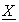must be decrypted to X = w0x+w1T1+ ---- +wmTm if E(Q, x*) and E(k, T1), ---, E(k, Tm) are correct. But if they are incorrect, P that does not know w0, w1, ---, wm cannot calculate X from.

Here, actually, E(Q, x*), E(Q, T1), ---, E(Q, Tm) in the above are vectors, therefore P obtains multiple relations about (m+1)-variables w0, w1, ---- wm, which may enable P to calculate w0, w2, ---- wm when m is small. But a slight extension disables P to calculate w0, w1, ---- wm even when m is small. This means P does not need to disclose numbers of plain and encryption forms pairs of test values. P does not need to disclose dummy terms of vector X and each test vector Tj either; in other words, verification of dummy terms has no meaning for V because they can have any values without making real terms inconsistent. Then, encryption function E(Q, x) can be protected from plain text attacks even in environments where correctness of numbers of encryption forms are verified.

### 3. Linear Mix-net

A scheme that enables authority A to calculate linear combinations of attribute values belong to same data holders without knowing correspondences between attribute values and their holders can be developed by linear Mix-nets as below . As mentioned before, one of advantages of linear Mix-nets is they use LE-based or MA-based encryption functions and can handle real numbers and integers efficiently totally in the same way.

Here, implementation of a re-encryption scheme based on LE-based encryption functions E(k1, x), E(k2, x), ---, E(kN, x) is straightforward, i.e. 1st mix-server M1 encrypts real number or integer x to z1-dimensional vector {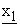(1),(2), ---,(z1)} based on its secret coefficient matrix {q1(i, j)} and dummy terms, and merges it and dummy elements {(z1+1),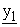(z1+2), ---,(z*1)} to construct z*1-dimensional vector E(k1, x) = {x1(1), x1(2), ---, x1(z*1)}. Then 2nd mix-server M2 adds dummy terms to {x1(1), ---, x1(z*1)} to construct z2-dimensional (z2 > z*1) vector {x1(1), ---, x1(z*1), x1(z*1+1), ---, x1(z2)}, encrypts it to {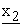(1),(2), ---,(z2)} by calculating each(s) as a linear combination of x1(1), ---, x1(z2) while using secret coefficient matrix {q2(i, j)}, and merges it and dummy elements {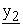(z2+1),(z2+2), ---,(z*2)} to construct z*2-dimensional vector E(k2, E(k1, x)) = {x2(1), x2(2), ---, x2(z*2)}. Remaining mix-servers behave in the same way.

In the remainder, a linear Mix-net is configured based on LE-based encryption functions, and notation E(kh*, x) is used to represent re-encryption form E(kh, E(kh-1, --- E(k1, x) --- )).

3.1. Configuration of LE-based Linear Mix-net

In LE-based linear Mix-net, mix-servers are arrayed also in the verification stage and numbers of mix-servers in the encryption and the decryption stages are not equal, i.e. it consists of data holders, authority A, mix-servers M1, ---, MT in the encryption and the verification stages and M1, ---, MN (N < T) in the decryption stage as shown in Figure 2. As same as in Figure 1 mix-servers in the encryption stage repeatedly encrypt individual attribute values, and based on the encryption results, authority A calculates encrypted weighted sums of individual data holders’ attribute values to be repeatedly decrypted by mix-servers in the decryption stage. But different form Figure 1 mix-servers in the encryption stage encrypt single attribute value XP(q) into multiple different forms, also before entering the decryption stage mix-servers in the verification stage decrypt individual encrypted attribute values to convince others of their honest encryptions.

Where, although each Mh in all stages discloses its encryption and decryption results publicly despite E(kh, x) is weak against plain text attacks to prove its correct handlings of attribute values, E(kh, x) is protected because Mh in each stage shuffles its encryption or decryption results. For an entity that does not know shuffling rules, every possible input and output values pair of Mh is a candidate of plain and encryption (or encryption and plain) forms pair of E(kh, x), and provided that Ω and Ψ are the number of data holders and the dimension of vector E(kh, x) respectively, ΩPΨ number of possibilities must be examined for obtaining Ψ-mutually independent plain and encryption forms pairs (ΩPΨ is greater than 101000 when Ω = 200 and Ψ = 100).

Figure 3 shows the data structure of data holder P’s q-th attribute value XP(q) that P puts in the encryption stage. Attribute ID and attribute parts correspond to q-th attribute name Iq (e.g. height of persons) and attribute value XP(q) itself (e.g. height of a particular person P). About copy ID part value d, P generates multiple copies for single XP(q) and d is the identifier of the d-th copy. The holder part value Zr(q, d)∙Rmod B is calculated by P from publicly known integer Zr(q, d)mod B as a used seal of P’s anonymous tag based credential S(P, R)  (B is a publicly known sufficiently large appropriate integer and notation mod B is omitted in the remainder).

In detail, for its q-th attribute value XP(q), P shows D-quadruplets E0(XP(q, d)) = {Iq, d, XP(q), Zr(q, d)∙R} (d =, 1, 2, ---, D) to 1st mix-server M1 to be encrypted repeatedly to quadruplets E1(XP(q, d)) = {Iq, d, E(k1, XP(q)d), Zr(q, d)∙R∙u(1)}, ---, EN(XP(q, d)) = {Iq, d, E(kN*, XP(q)d), Zr(q, d)∙R∙u*(N)}, ---, ET(XP(q, d)) = {Iq, d, E(kT*, XP(q)d), Zr(q, d)∙R∙u*(T)}, (d =, 1, 2, ---, D) by M1, ---, MN, ---, MT in the encryption stage, where E(kh*, XP(q)d) represents a re-encryption form of XP(q) that is generated based on dummy terms and dummy elements corresponding to copy ID value d, therefore although E(kh*, XP(q)1), ---, E(kh*, XP(q)D) are decrypted to same value XP(q) they have different forms.

About the verification stage, authority A collects quadruplets ET(XP(q, 1)), ---, ET(XP(q, D)), which are calculated from E0(XP(q, 1)), ---, E0(XP(q, D)) in the encryption stage corresponding to attribute value XP(q), to construct single triplet ET(XP(q)) = {Iq, E(kT*, XP(q)*), Zr(q)∙R∙u*(T)w(A)} that has the same structure as in Figure 3 except it does not include the copy ID part. Then, MT, ---, M1 decrypt ET(XP(q)) repeatedly to triplets ET-1(XP(q)), ---, E0(XP(q)). Important things are firstly E(kT*, XP(q)*) in triplet ET(XP(q)) is decrypted also to XP(q), and secondly, each mix-server Mh cannot identify the correspondence between Eh(XP(q, d)) in the encryption stage and Eh(XP(q)) in the verification stage for each d, in other words, attribute and holder parts values in triplets ET(XP(q)), ---, E1(XP(q)) have different forms from those in quadruplets ET(XP(q, d)), ---, E1(XP(q, d)).

Finally, the data structure of the weighted sum of P’s attribute values X(P) = a1XP(1)+ --- +aQXP(Q) put in the decryption stage consists of attribute part and holder part values pair EN(X(P)) = {E(kN*, X(P)), ZR∙u*(N)r*}. Its attribute part and holder part values are aggregations of those in 1st copies of re-encrypted quadruplets EN(XP(1, 1)), ---, EN(XP(Q, 1)) in the encryption stage, i.e. E(kN*, X(P)) = a1E(kN*, XP(1)1)+ --- +aQE(kN*, XP(Q)1) and ZR∙u*(N)∙r* = ZR∙u*(N)r(1, 1)r(2, 1)---r(Q, 1). Here, it must be noted that authority A generates EN(X(P)) from EN(XP(1, 1)), ---, EN(XP(Q, 1)) instead of ET(XP(1, 1)), ---, ET(XP(Q, 1)).

Under the above settings, first 3 difficulties in Introduction are removed by anonymous credential S(P, R) and holder part values of quadruplets in the encryption stage and pairs in the decryption stage. In detail, to register itself as an authorized entity, each data holder P shows its exact identity to authority A, and A issues integers Zr(1, d), ---, Zr(Q, d) (d = 1, 2, ---, D) and anonymous credential S(P, R) that includes P’s secret unique integer R to P. After that, P generates secret integer y(P, q), calculates S(P, R)y(P, q), and shows S(P, R)y(P, q) together with XP(q) to 1st mix-server M1 in the encryption stage. At the same time, P calculates holder part value Zr(q, d)∙R from Zr(q, d) for each d as a used seal of S(P, R) to be incorporated in each quadruplet E0(XP(q, d)) = {Iq, d, XP(q), Zr(q, d)∙R}, where, anonymous credential S(P, R) forces P to honestly calculate holder part value Zr(q, d)∙R from Zr(q, d) by using secret integer R in S(P, R). About integers Z and r(q, d), Z is common to all attribute vales of all data holders and publicly known, on the other hand, r(q, d) is unique to q-th attribute values for each d and it is secret from all entities including A and mix-servers.

Then the 1st difficulty is removed, i.e. anonymous credential S(P, R) enables P to convince M1 of its eligibility without disclosing its identity or secret integer R . Here, P assigns different values to secret integers y(P, 1), ---, y(P, Q), also each holder part value Zr(q, d)∙R is constructed by integers Z, r(q, d) and R with the above properties. Therefore entities other than P cannot identify links among P’s attribute values XP(1), ---, XP(Q) even if they examine credential forms S(P, R)y(P, 1), ---, S(P, R)y(P, Q) or used seals Zr(1, d)∙R, ---, Zr(Q, d)∙R. When difficulties of solving discrete logarithm problems are considered, to know that the above credential forms or used seals are calculated from same S(P, R) or R is computationally infeasible for entities that do not know y(P, q), R or r(q, d).

About the 2nd and the 3rd difficulties, holder part value Zr(q, d)∙R in initial quadruplet E0(XP(q, d)) is transformed to Zr(q, d)∙R∙u(1)u(2)---u(N) = Zr(q, d)∙R∙u*(N) by M1, ---, MN in the encryption stage as a holder part value of re-encrypted quadruplet EN(XP(q, d)), and before entering the decryption stage, A asks mix-servers to calculate (Zr(q, 1)∙R∙u*(N))r(1, 1)∙r(2, 1)---r(q-1, 1)∙r(q+1, 1)---r(Q, 1) = ZR∙u*(N)r(1, 1)r(2, 1)---r(Q, 1) = ZR∙u*(N)r* from Zr(q, 1)∙R∙u*(N) for each q. Therefore, value ZR∙u*(N)r* becomes common to P’s all encrypted quadruplets EN(XP(1, 1)), ---, EN(XP(Q, 1)), and as a result, to calculate pair EN(X(P)) = {E(k*, X(P)), ZR∙u*(N)r*} A can collect EN(XP(1, 1)), ---, EN(XP(Q, 1)) despite that M1, ---, MN shuffle their encryption results. Here, u(h) is mix-server Mh’s secret integer common to all attribute values of all data holders, and although no one knows each r(q, d) mix-servers can calculate ZR∙u*(N)r* from Zr(q, 1)∙R∙u*(N) as in Sec. 3.2.3.

In the same way, A can collect all quadruplets ET(XP(q, 1)), ---, ET(XP(q, D)) corresponding to XP(q) to construct triplet ET(XP(q)) to be decrypted in the verification stage. Also, P can identify finally decrypted pair E*0(X(P)) = {X(P), ZR∙u*(N)r*v*} in the decryption stage based on its holder part value ZR∙u*(N)∙r*∙v*, i.e. provided that mix-servers calculate Zu*(N)∙r*∙v* separately, only P that knows R can calculate ZR∙u*(N)∙r*∙v* in pair E*0(X(P)) from Zu*(N)∙r*∙v*. Moreover although R is P’s secret, P must calculate it honestly as a used seal of its credential S(P, R).

In the above, unique integer r(q, d) secret from all entities can be generated easily. In detail, each Mh generates its secret integer r(q, d; h) and calculates Zr(q, d; 1)∙r(q, d; 2)---r(q, d; h-1)∙r(q, d; h) from Zr(q, d; 1)∙r(q, d; 2)---r(q, d; h-1) received from Mh-1 to forward the result to Mh+1 so that finally MT can calculate Zr(q, d; 1)∙r(q, d; 2)---r(q, d; T) = Zr(q, d). Namely, no one knows all r(q, d; 1), ---, r(q, d; T) and calculating r(q, d) from Zr(q, d) is a discrete logarithm problem. Also uniqueness of r(q, d) can be maintained by discarding r(q, d) to replace it with new one when mix-servers had calculated same value Zr(q, d) before. Integers u*(N), v* and r* are generated in the same way. Therefore, no one can know values of r(q, d), u*(N), v* or r*, and as a result, anyone including P itself cannot examine holder part values to know the correspondence between P and encrypted quadruplet EN(XP(q, d)) or between finally decrypted pair E*0(X(P)) and each EN(XP(q, d)).

LE-based encryption functions and the verification stage remove the remaining difficulties. Firstly, encryption function E(kN*, x) is additive because each E(kh, x) is LE-based. Therefore, authority A can calculate encryption form E(kN*, a1XP(1)+a2XP(2)+ --- +aQXP(Q)) from encrypted attribute values E(kN*, XP(1)1), E(kN*, XP(2)1), ---, E(kN*, XP(Q)1) as E(kN*, a1XP(1)+ --- +aQXP(Q)) = E(kN*, XP(1)1)+ --- +E(kN*, XP(Q)1). Namely, the 4th difficulty is removed if function f(x1, ---, xQ) is a linear combination of attribute values x1, ---, xQ.

About the 5th difficulty, mix-servers in the encryption and the verification stages transform each attribute value in different ways, i.e. corresponding to same attribute value XP(q), mix-server Mh in the encryption stage calculates quadruplet Eh(XP(q, d)), and Mh+1 in the verification stage calculates triplet Eh(XP(q)) so that no one can identify the correspondence between them. Therefore, if initial quadruplet E0(XP(q, d)) was dishonestly transformed to Eh(X*P(q, d)) by Mh in the encryption stage, even Mh in the verification stage cannot replace E(kh*, X*P(q)*) in Eh(X*P(q)) with E(kh*, XP(q)*) that is finally decrypted to XP(q), because it does not know triplet Eh(X*P(q)) corresponding to Eh(X*P(q, d)). This means authority A can verify the correct encryption of each E0(XP(q, d)) by comparing XP(q) in it and X*P(q) in decrypted triplet E0(X*P(q)) in the verification stage, i.e. EN(X*P(q)) is incorrect when XP(q) ≠ X*P(q). Here, data holder P can identify triplet E0(X*P(q)) corresponds to it in the same way as it finds pair E*0(X(P)) in the decryption stage.

By exploiting, integers Zr(1, d), ---, Zr(Q, d), verifiable features of LE-based encryption functions and features of anonymous tag based credentials, A and data holders also can detect dishonesties in the decryption stage, identify liable entities, and re-calculate correct results without knowing secrets of honest entities, i.e. the 5th difficulty is removed.

3.2. Behaviours of the LE-based Linear Mix-net

After quadruplets E0(XP(q, d)) = {Iq, d, XP(q), Zr(q, d)∙R} (d = 1, 2, ---, D) were disclosed publicly corresponding to attribute value XP(q) of anonymous data holder P, individual mix-servers and authority A behave as below. In the remainder, u*(h), v*(h) and w*(h) represent products u(1)u(2)---u(h), v(N)v(N-1)---v(h) and w(A)w(N)w(N-1)---w(h), and as a special case v* = v*(1) and w* = w*(1). Where, u(h), v(h) and w(h) are integers common to all attribute values of all data holders and secrets of h-th mix-server Mh, and w(A) is a secret integer of authority A and common to all attribute values of all data holders.

3.2.1. Encryption Stage

1st mix-server M1 in the encryption stage that picks disclosed E0(XP(q, d)) = {Iq, d, XP(q), Zr(q, d)∙R} encrypts XP(q) to E(k1, XP(q)d) by encryption key k1, calculates Mr(q, d)∙R∙u(1) from Mr(q, d)∙R by using secret integer u(1), and constructs quadruplet E1(XP(q, d)) = {Iq, d, E(k1, XP(q)d), Zr(q, d)∙R∙u(1)}. Other mix-servers behave in the same way, i.e. each Mh picks Eh-1(XP(q, d)) = {Iq, d, E(k(h-1)*, XP(q)d), Zr(q, d)∙R∙u*(h-1)} disclosed by Mh-1, encrypts E(k(h-1)*, XP(q)d) to E(kh*, XP(q)d) and calculates Zr(q, d)∙R∙u*(h) from Zr(q, d)∙R∙u*(h-1) by its secret key kh and secret integer u(h), and constructs Eh(XP(q, d)) = {Iq, d, E(kh*, XP(q)d), Zr(q, d)∙R∙u*(h)} to disclose it publicly. As a result, E0(XP(q, d)) received by M1 is finally transformed to EN(XP(q, d)) and ET(XP(q, d)) by MN and MT respectively, where each Mh shuffles its generating quadruplets of course.

Then, because no one knows all keys k1, ---, kN , ---, kT nor integers u(1), ---, n(N), ---, u(T), anyone cannot link E0(XP(q, d)) to EN(XP(q, d)) or ET(XP(q, d)) unless all mix-servers conspire. Anyone except P cannot know links among P’s attribute values XP(1), XP(2), ---, XP(Q) either. About encryption function E(kh, x), although each Mh shuffles its encryption results, anyone can obtain a plain and encryption forms pair of E(kh, x). Namely, if an entity calculates Xh-1 and Xh as sums of all attribute part values in quadruplets that Mh receives and generates respectively, {Xh-1, Xh} is a plain and encryption forms pair because E(kh, x) is additive. But, Mh can protect E(kh, x) from plaintext attacks because known pair is only {Xh-1, Xh}.

3.2.2. Verification Stage

After the encryption stage, authority A conducts the verification stage to examine whether individual quadruplets were honestly encrypted or not, and when dishonestly handled quadruplets are detected it asks mix-servers to carry out the encryption stage again while using new secret values including encryption keys. Also, A identifies dishonest mix-servers if necessary to replace them with new ones as will be discussed in Sec. 3.4.2. Therefore, the encryption stage eventually generates correct encryption results.

To examine individual quadruplets, firstly authority A collects ET(XP(q, 1)) = {Iq, 1, E(kT*, XP(q)1), Zr(q, 1)∙R∙u*(T)}, ---, ET(XP(q, D)) = {Iq, D, E(kT*, XP(q)D), Zr(q, D)∙R∙u*(T)} corresponding to each attribute value XP(q) of each data holder P. Here, provided that r(q,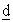; h) and r(q) represent products r(q, 1; h)∙r(q, 2; h)---r(q, d-1; h)∙r(q, d+1; h)---r(q, D; h) and r(q, 1)∙r(q, 2)---r(q, D) respectively, each Mh receivesfrom Mh-1 and calculatesby using its secret integer r(q,, h) to forward it to Mh+1. As a result, MT calculates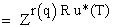. Therefore, A can collect EN(XP(q, 1)), ---, EN(XP(q, D)) that includeas their holder part values.

After that, each Mh (h ≤ Y < N) calculates dh1E(kT*, XP(q)1)+ --- +dhDE(kT*, XP(q)D)+E(kT*, 0h(P, q)) = E(kT*, dh1XP(q)1+ --- +dhDXP(q)D+0h(P, q)) = E(kT*, XP(q; h)), and A calculates {E(kT*, XP(q; 1))+ --- +E(kT*, XP(q; Y))}/Y = E(kT*, XP(q)*). Here, dh1, dh2, ---, dhD are real numbers secrets of Mh and relation dh1+ --- +dhD = 1 holds, and E(kT*, 0h(P, q)) is Mh’s secret encryption form of value 0. Therefore each E(kT*, XP(q; h)) is decrypted to XP(q). In addition, E(kT*, XP(q)*) is also represented as E(kT*, XP(q)*) = E(kT*, d1XP(q)1+ --- +dDXP(q)D+0*(P, q)) for some real number coefficients d1, ---, dD that satisfy d1+ --- +dD = 1 (where E(kT*, 0*(P, q)) = {E(kT*, 01(P, q)+ --- +0Y(P, q))}/Y), and this means E(kT*, XP(q)*) is also decrypted to XP(q). But no one knows coefficients d1, ---, dD or encryption form E(kT*, 0*(P, q)) because dh1, dh2, ---, dhD and E(kT*, 0h(P, q)) are known only to Mh.

About encryption form E(kT*, 0h(P, q)), each Mh can generate it by choosing data holders P(h1), ---, P(hS), attribute IDs Iq(h1), ---, Iq(hS) and copy IDs pairs {d(h1),}, ---, {d(hS),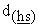} arbitrarily as its secrets and linearly combiningby its secret coefficients, i.e. E(kT*, XP(hs)(q(hs))d(hs)) and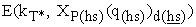are encryption forms of same value XP(hs)(q(hs)). Mh also can identify pair {E(kT*, XP(hs)(q(hs))d(hs)),} because they are accompanied by same holder part value Zr(q(hs))∙R(hs)∙u*(T).

Then A generates secret integer w(A), and constructs triplet ET(XP(q)) = {Iq, E(kT*, XP(q)*), Zr(q)∙R∙u*(T)∙w(A)} to disclose it publicly, and mix-servers MT, ---, M1 in the verification stage repeatedly decrypt ET(XP(q)) to E0(XP(q)) = {Iq, XP(q), Zr(q)∙R∙u*(T)∙w*}. In detail, each Mh picks Eh(XP(q)) = {Iq, E(kh*, XP(q)*), Zr(q)∙R∙u*(T)∙w*(h+1)} disclosed by Mh+1, decrypts E(kh*, XP(q)*) to E(k(h-1)*, XP(q)*) calculates Zr(q)∙R∙u*(T)∙w*(h) = Zr(q)∙R∙u*(T)∙w*(h+1)∙w(h) by using key kh-1 and integer w(h), constructs triplet Eh-1(XP(q)) = {Iq, E(k(h-1)*, XP(q)*), Zr(q)∙R∙u*(T)∙w*(h)}, and discloses it to be picked by Mh-1. As a consequence, M1 generates decrypted triplet E0(XP(q)) = {Iq, XP(q), Zr(q)∙R∙u*(T)∙w*}.

Here, although A and MN, ---, M1 shuffle their calculation results, links among copies of quadruplets ET(XP(q, 1)), ---, ET(XP(q, D)) are revealed. Nevertheless, P can preserve its privacy, i.e. they are encryption forms of same attribute value XP(q). Also, mix-servers can protect their encryption functions from plain text attacks despite anyone can obtain plain and encryption forms pair {0, E(kT*, 0)} as above, i.e. no one knows its dummy term values.

<Detecting dishonesties in the encryption stage>

In the above, because no one knows coefficients d1, ---, dD, encryption form E(kh*, 0*(P, q)) or integer w*(h), anyone cannot link triplet Eh(XP(q)) = {Iq, E(kh*, XP(q)*), Zr(q)∙R∙u*(T)∙w*(h+1)} to corresponding quadruplet Eh(XP(q, d)) = {Iq, d, E(kh*, XP(q)d), Zr(q, d)∙R∙u*(h)}. This means if initial quadruplet E0(XP(q, d)) is dishonestly encrypted to ET(X*P(q, d)) in the encryption stage, any Mh in the verification stage cannot modify corresponding triplet Eh(X*P(q)) to Eh(XP(q)) so that it is finally decrypted to E0(XP(q)) = {Iq, XP(q), Zr(q)∙R∙u*(T)∙w*} (actually, 1st mix-server M1 can do as will be discussed later).

Authority A detects dishonesties in the encryption stage by using this property. In detail, firstly A requests mix-servers to calculate integer Zr(q)∙u*(T)∙w* for each q to disclose it publicly. After that for each q, each data holder P calculates used seals Zr(q, 1)∙R and Zr(q)∙R∙u*(T)∙w* of its credential S(P, R) from Zr(q, 1) and Zr(q)∙u*(T)∙w*, and based on Zr(q, 1)∙R and Zr(q)∙R∙u*(T)∙w* finds E0(XP(q, 1)), i.e. 1st copy of the initial quadruplet corresponding to attribute value XP(q), and triplets E0(X*P(q)) in the verification stage.

Then, P shows initial quadruplet and decrypted triplet pair <E0(XP(q, 1)), E0(X*P(1))> to A while convincing A of its ownership of the pair by used seals Zr(q, 1)∙R and Zr(q)∙R∙u*(T)∙w*, and A determines pair <E0(XP(q, 1)) = {Iq, 1, XP(q), Zr(q, 1)∙R}, E0(X*P(q)) = {Iq, X*P(q), Zr(q)∙R∙u*(T)∙w*}> is inconsistent, i.e. at least one copy E0(XP(q, d)) was dishonestly handled, when XP(q) ≠X*P(q). Here, correctness of XP(q) in E0(XP(q, 1)) is ensured because P shows XP(q) to M1 in its plain form. About privacy preservation, P must report pairs separately and without disclosing its identity of course.

A also can force all data holders to report their all pairs, i.e. when no one appears as the holder of pair <E0(XP(q, 1)), E0(XP(q))>, it asks all data holders to calculate used seals of their credentials from Zr(q, 1) and Zr(q)∙u*(T)∙w* while disclosing their identities as will be discussed in Sec. 3.4.3. In a case where mix-servers transform holder part value Zr(q, d)∙R in E0(XP(q, d)) to an invalid value P cannot identify its decrypted triplet in the verification stage, but even in this case A can detect the dishonestly handled quadruplet as E0(XP(q, 1)) that is not paired with any triplet.

In the above, mix-servers M1, ---, MT can calculate Zr(q)∙u*(T)∙w* as same as Zr(q)∙R∙u*(T) at the beginning of this subsection. Namely provided that r(q; h) represents product r(q, 1; h)∙r(q, 2; h)---r(q, D; h), each Mh calculates Z{r(q; 1)∙r(q; 2)----r(q; h-1)r(q; h)}{u(1)∙u(2)----u(h-1)u(h)}{w(A)∙w(1)∙w(2)----w(h-1)w(h)} from Z{r(q; 1)----r(q; h-1)}{u(1)----u(h-1)}{w(A)∙w(1)----w(h-1)} given by Mh-1 to forward it to Mh+1 so that finally MT calculates Z{r(q; 1)----r(q; T)}{u(1)----u(T)}{w(A)∙w(1)----w(T)} = Zr(q)∙u*(T)∙w*. Here, although P knows integer R, it cannot identify triplet Eh(XP(q)) = {Iq, E(kh*, XP(q)*), Zr(q)∙R∙u*(T)∙w*(h+1)} based on Z{r(q; 1)----r(q; h)}{u(1)----u(h)}{w(A)∙w(1)----w(h)} disclosed by Mh for h > 1, because r(q; 1)----r(q; h)u(1)----u(h)w(A)w(1)----w(h) and r(q)u*(T)w*(h+1) are different.

About privacy of data holder P, P is anonymous, and because it reports pairs <E0(XP(1, 1)), E0(XP(1))>, ---, <E0(XP(Q, 1)), E0(XP(Q))> separately, anyone other than P cannot know links among XP(1), ---, XP(Q). Although E0(XP(q)) includes plain attribute value XP(q), XP(q) is publicly known from the beginning.

<Dishonest 1st mix-server M1>

1st mix-server M1 in the encryption stage can encrypt E0(XP(q, d)) dishonestly while making triplet E0(XP(q)) in the verification stage include consistent attribute value XP(q). For example, provided that X*P(q) is a unique value, even if M1 dishonestly encrypted E0(XP(q, d)) = {Iq, d, XP(q), Zr(q, d)∙R} to E1(X*P(q, d))= {Iq, d, E(k1, X*P(q)), Zr(q, d)∙R∙u(1)} in the encryption stage for each d, because X*P(q) is unique it can identify incorrect triplet E0(X*P(q)) = {Iq, X*P(q), Zr(q)∙R∙u*(T)∙w*} in the verification stage and replace X*P(q) in it with XP(q) to produce correct decrypted triplet E0(XP(q)) = {Iq, XP(q), Zr(q)∙R∙u*(T)∙w*}.

To disable above dishonesties, in other words, to convince others that M1 is honest, after completing the verification stage, A discloses its secret integer w(A) and asks mix-servers M1, M2, ---, MY (Y < N) to disclose their encryption keys k1, k2, ---, kY, and secret integers u(1), u(2), ----, u(Y), w(1), W(2), ---, w(Y). Namely, by the disclosed information, anyone can confirm M1 is honest. On the other hand, encryption keys kY+1, ---, kN, ---, kT and integers u(Y+1), ---, u(N), ---, u(T), w(Y+1), ---, w(N), ---, w(T) are still secrets of MY+1, ---, MT, therefore secrets of honest data holders can be preserved. Also, A and mix-servers replace secret integers w(A), u(1), ---, u(T), w(1), ---, w(T) and encryption keys k1, ---, kT with new ones for handling new sets of attribute values.

About last mix-server MT, if it conspires with authority A, it also can encrypt ET-1(XP(q, d)) to ET(X*P(q, d)) dishonestly and decrypt ET(X*P(q)) to ET-1(XP(q)) that is finally decrypted to correct value XP(q), but incorrect ET(X*P(q, d)) does not affect the final calculation results because MN+1, MN+2, ---, MT are not involved in the decryption stage.

3.2.3. Calculating Encrypted Weighted Sums of Attribute Values

To calculate encrypted weighted sums of attribute values corresponding to individual data holders, provided that rq(h) and r* represent products {r(1, 1; h)∙r(2, 1; h)--- r(q-1, 1; h)∙r(q+1, 1; h)---r(Q, 1; h)} and {r(1, 1)∙r(2, 1)---r(Q, 1)} respectively, authority A asks mix-servers M1, ---, MT to transform the holder part value of each quadruplet EN(XP(q, 1)) = {Iq, 1, E(kN*, XP(q)1), Zr(q, 1)∙R∙u*(N)} from Zr(q, 1)∙R∙u*(N) to ZR∙u*(N)∙r*, i.e. each Mh calculates Zr(q, 1)∙R∙u*(N)rq(1)∙rq(2)---rq(h-1)rq(h) from Zr(q, 1)∙R∙u*(N)rq(1)∙rq(2)--- rq(h-1) received from Mh-1 to forward the result to Mh+1. Therefore, finally MT calculates Zr(q, 1)∙R∙u*(N)rq(1)---rq(T) = ZR∙u*(N)r(1, 1)---r(Q, 1) = ZR∙u*(N)r*, and same value ZR∙u*(N)∙r* is assigned to P’s quadruplets EN(XP(1, 1)), ---, EN(XP(Q, 1)) as their holder part values. Then, A can collect EN(XP(1, 1)), ---, EN(XP(Q, 1)) from all quadruplets disclosed by MN to calculate the encrypted weighted sum of P’s attribute values as E(kN*, X(P)) = E(kN*, a1XP(1)1+ --- +aQXP(Q)1) = a1E(kN*, XP(1)1)+ --- +aQE(kN*, XP(Q)1) and to construct pair E*N(X(P)) = {E(kN*, X(P)), ZR∙u*∙r*}.

3.2.4. Decryption Stage

In the decryption stage, mix-servers MN, MN-1, ---, M1 repeatedly decrypt pair E*N(X(P)) to E*N-1(X(P)) = {E(k(N-1)*, X(P)), ZR∙u*(N)∙r*∙v(N)}, E*N-2(X(P)) = {E(k(N-2)*, X(P)), ZR∙u*(N)∙r*∙v*(N-1)}, ---, E*0(X(P)) = {X(P), ZR∙u*(N)∙r*∙v*}. Here, although each Mh shuffles its decryption results A can verify correct decryptions of E*N(X(P)) partially without knowing secrets of mix-servers by exploiting additive feature of each E(kh, x). Namely, if A calculates sums of attribute part values in all pairs received and generated by Mh as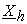and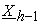respectively, bothandmust be encryption forms of, weighted sum of all attribute values of all data holders. This means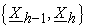is a plain and encryption forms pair of E(kh, x), and additive (as a result verifiable) feature of E(kh, x) enables A to force mix-servers to decrypt pairs so that sums of their decrypted attribute part values coincide witheven if they decrypt individual pairs dishonestly.

About encryption function E(kh, x) of each mix-server Mh, because Mh shuffles its decryption results, no one other than Mh can identify plain and encryption forms pair {E(k(h-1)*, X(P)), E(kh*, X(P))} except the above pair. Therefore, Mh can protect E(kh, x) from plain text attacks also in the decryption stage.

Nevertheless, data holder P can find its pair E*0(X(P)) = {X(P), ZR∙u*(N)∙r*∙v*} to take actions for the pair. Firstly, A asks mix-servers to calculate Zu*(N)∙r*∙v* in the same way as in Sec. 3.2.3. Then, each P calculates (Zu*(N)∙r*∙v*)R = ZR∙u*(N)∙r*∙v* from Zu*(N)∙r*∙v* based on integer R in its credential S(P, R), finds pair E*0(X(P)) according to ZR∙u*(N)∙r*∙v*, and convinces A of its ownership of E*0(X(P)). Where, although P is anonymous, A can confirm P’s ownership of pair E*0(X(P)), because P must calculate ZR∙u*(N)∙r*∙v* honestly as a used seal of S(P, R).

3.3. Detecting Dishonesties

In the encryption stage, data holder P puts its initial quadruplet E0(XP(q, d)) = {Iq, d, XP(q), Zr(q, d)∙R} honestly, because P shows XP(q) in its plain form and it calculates Zr(q, d)∙R from Zr(q, d) as a used seal of its credential. This means P cannot behave dishonestly in any stage. Also, the verification stage ensures that mix-servers in the encryption stage eventually generate correct encryption results. Therefore, remaining dishonesties to be detected are ones in the decryption stage, i.e. each mix-server Mh may replace elements of pair E*h(X(P)) with those of other pair E*h(X(P*)) and may simply decrypt E*h(X(P)) incorrectly.

Provided that volume of duties P must accomplish increases (or decreases) when weighted sum of P’s attribute values X(P) increases, authority A can easily detect above dishonesties as below. Namely as mentioned in the previous subsection, each mix-server Mh in the decryption stage must decrypt each pair E*h(X(P)) it receives from Mh+1 so that, sum of attribute part values in all pairs it generates, is finally decrypted to, the weighted sum of attribute values of all data holders. Therefore, if dishonesties bring any benefit to a data holder some other data holder necessarily suffers loss. For example, if X(P*) is the amount data holder P* must pay, when X(P*) becomes less than the actual value, for at least one other data holder P, weighted sum of its attribute values X(P) becomes larger than the actual value. As a result, P claims decrypted pair E*0(X(P)) is incorrect. It is also possible to endow each P with the ability to automatically notice A that E*0(X(P)) is incorrect by distributing adequate computer programs to data holders.

Also, each data holder P must appear to take actions for E*0(X(P)) as will be discussed in Sec. 3.4.3, although X(P) in it may not be correct. Here, Mh may transform a holder part value of E*h(X(P)) = {E(kh*, X(P)), ZR∙u*(N)∙r*∙v(h+1)} in the decryption stage to an invalid value or to the one corresponding to data holder P* different from P. But decrypted pairs accompanied by invalid holder part values are detected as the ones of which holders cannot be identified by the procedure in Sec. 3.4.3. In the latter case, some data holders claim that weighted sums of their attribute values are incorrect.

3.4. Identifying Dishonest Entities
3.4.1. Dishonest Mix-servers in the Decryption Stage

When authority A determines some decrypted pairs include invalid holder part values or some data holders claim that decrypted pairs corresponding to them are incorrect, A can identify liable entities and re-calculate correct pairs without knowing secrets of honest entities. In the following, pair E*0(X*(P)) = {X*(P), ZR∙u*(N)∙r*∙v*} in the decryption stage is assumed incorrect, i.e. it is a pair that includes an invalid holder part value or that is claimed as inconsistent by anonymous data holder P.

To identify mix-servers liable for each incorrect pair E*0(X*(P)), authority A requests mix-servers M1, ---, MN to prove their correct decryptions. In detail, M1 finds pair E*1(X*(P)) = {E(k1, X*(P)), ZR∙u*(N)∙r*∙v*(2)} that corresponds to E*0(X*(P)), and A confirms that M1 certainly had received E*1(X*(P)) from M2 in the decryption stage and E*0(X*(P)) is the correct decryption form of E*1(X*(P)). In the same way, each Mh finds pair E*h(X*(P)) = {E(kh*, X*(P)), ZR∙u*(N)∙r*∙v*(h+1)} corresponds to E*h-1(X*(P)) = {E(k(h-1)*, X*(P)), ZR∙u*(N)∙r*∙v*(h)} forwarded by Mh-1, and A confirms Mh certainly had received E*h(X*(P)) from Mh+1 and E*h-1(X*(P)) is the correct decryption form of E*h(X*(P)). Namely, Mh is dishonest when E*h(X(P)) that is decrypted to E*h-1(X*(P)) does not exist.

Here, A can examine the consistency of pair <E*h(X*(P)), E*h-1(X*(P))> without knowing secrets of Mh. Namely, consistency of attribute part values E(kh*, X*(P)) and E(k(h-1)*, X*(P)) can be verified because E(kh, x) is additive and verifiable (moreover, encryption keys of M1, ---, MY are disclosed as in Sec. 3.2.2). About holder part values, pair {ZR∙u*(N)∙r*∙v*(h+1), ZR∙u*(N)∙r*∙v*(h)} is consistent if ZR∙u*(N)∙r*∙v*(h) is calculated from ZR∙u*(N)∙r*∙v*(h+1) by using Mh’s secret integer v(h) that is common to all attribute values of all data holders. Therefore, when A calculates product of holder part values in all pairs Mh had generated in the decryption stage as Z*R*∙u*(N)∙r*∙v*(h) for each h and defines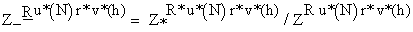, along the scheme of Diffie and Hellman , it can determine pair {ZR∙u*(N)∙r*∙v*(h+1), ZR∙u*(N)∙r*∙v*(h)} is consistent if ZR∙u*(N)∙r*∙v*(h) andare calculated as (ZR∙u*(N)∙r*∙v*(h+1))v(h) andby same unknown integer v(h).

In the following notations ZP(h+1), Z-(h+1), ZP(h) and Z-(h) represent ZR∙u*(N)∙r*∙v*(h+1),, ZR∙u*(N)∙r*∙v*(h) and Z-R∙u*(N)∙r*∙v*(h) respectively, therefore pair {ZR∙u*(N)∙r*∙v*(h+1), ZR∙u*(N)∙r*∙v*(h)} is consistent if relations ZP(h+1)v(h) = ZP(h) and Z-(h+1)v(h) = Z-(h) hold for same unknown v(h). Also it must be noted that ZP(h+1)Z-(h+1) = Z*R*∙u*(N)∙r*∙v*(h+1). Then, to confirm relations ZP(h+1)v(h) = ZP(h) and Z-(h+1)v(h) = Z-(h), A generates its secret integers δ1, δ2, δ3, calculates pairs {ZP(h+1)δ1, ZP(h)δ1}, {Z-(h+1)δ2, Z-(h)δ2}, {(ZP(h+1)Z-(h+1))δ3, (ZP(h)Z-(h))δ3}, and shows ZP(h+1)δ1, Z-(h+1)δ2, (ZP(h+1)Z-(h+1))δ3 to Mh. After that Mh calculates (ZP(h+1)δ1)v(h), (Z-(h+1)δ2)v(h), {(ZP(h+1)Z-(h+1))δ3}v(h) from them and its secret integer v(h), and finally A confirms that ZP(h) and Z-(h) were calculated by same v(h) if relations (ZP(h+1)δ1)v(h) = ZP(h)δ1, (Z-(h+1)δ2)v(h) = Z-(h)δ2 and {(ZP(h+1)Z-(h+1))δ3}v(h) = {ZP(h)Z-(h)}δ3 hold.

In the above, even if Mh had calculated ZP(h) and Z-(h) as ZP(h+1)λ and Z-(h+1)v(h) respectively while using different integers λ and v(h), it still can satisfy relations (ZP(h+1)δ1)λ = ZP(h)δ1 and (Z-(h+1)δ2)v(h) = Z-(h)δ2 despite it does not know δ1 or δ2. But according to the difficulty of solving discrete logarithm problems, it cannot find integer λ* that satisfies relation {(ZP(h+1)(Z-(h+1))δ3}λ* = (ZP(h)Z-(h))δ3 = (ZP(h+1)λZ-(h+1)v(h))δ3, because it cannot represent ZP(h+1) or Z-(h+1) as a function of Z-(h+1) or ZP(h+1) respectively.

Then, once Mh was identified as dishonest, Mh, ---, M1 must honestly decrypt E*h(X(P)) to generate correct decrypted pair E*0(X(P)) = {X(P), ZR∙u*(N)∙r*∙v*} (because A can verify correct decryption of Mh as above). About data holder P, it can conceal the correspondence between X(P) and it because it is still anonymous. But it must be noted that to protect E(kh, x) from plain text attacks (in other words not to disclose many plain and encryption forms pairs) A can examine only predefined number of incorrect decrypted pairs even if many decrypted pairs are determined as incorrect as will be discussed in Sec. 3.4.2. In addition, in cases where last mix-server MN is dishonest, incorrect pair <E*N(X(P)), E*N-1(X*(P))> is disclosed, and corresponding data holder P may obtain plain and encryption forms pairs {XP(1), E(kN*, XP(1)1)}, ---, {XP(Q), E(kN*, XP(Q)1)}, i.e. P knows its attribute values XP(1), ---, XP(Q) and can know encrypted quadruplets EN(XP(1, 1)), ---, EN(XP(Q, 1)) as A collects them to calculate E*N(X(P)). Despite E(k1, x), ---, E(kN, x) are weak against plain text attacks, still they can be protected because the number of disclosed pairs is not large except cases where many attribute values are assigned to each data holder. But when individual data holders have many attribute values A must carry out all stages again as below.

Namely, when A cannot identify dishonest mix-servers among M1, M2, ---, MU (U < N), it conducts all stages again from the encryption stage while arraying mix-servers in the different order so that MU+1, MU+2, ---, MN are allocated before M1, M2, ---, MU in the encryption stage. Then, if MN behaves dishonestly again A can identify it without worrying about the disclosure of numbers of pairs {XP(1), E(kN*, XP(1)1)}, ---, {XP(Q), E(kN*, XP(Q)1)}. Fortunately, usually authority A or mix-servers do not behave dishonestly, because they are not anonymous, their dishonesties are necessarily revealed and they cannot continue their businesses after their dishonesties are revealed. This means that in actual applications authority A can conduct all stages again from the start without degrading the performance. Another fortunate thing is data holders are not required to put their attribute values again to re-conduct individual stages.

3.4.2. Dishonest Mix-servers in the encryption and the verification stages

As in Sec. 3.2.2, authority A conducts the encryption stage again when initial quadruplet E0(XP(q, 1)) is not paired with any triplet in the verification stage or some data holder P claims pair <E0(XP(q, 1)), E0(X*P(q))> is inconsistent. But if A wants to remove dishonest mix-servers or replace them with new ones, it must identify dishonest mix-servers. A can identify dishonest mix-servers in the encryption and the verification stages without knowing secrets of honest entities as below.

Provided that pair <E0(XP(q, 1)), E0(X*P(q))> is inconsistent or E0(XP(q, 1)) is not accompanied by any triplet, to identify dishonest mix-servers, firstly 1st mix-server M1 encrypts E0(XP(q, 1)), ---, E0(XP(q, D)) to E1(XP(q, 1)), ---, E1(XP(q, D)) by using secret parameters that it had used in the encryption stage. In the same way, each Mh encrypts quadruplets Eh-1(XP(q, 1)), ---, Eh-1(XP(q, D) forwarded by Mh-1 to Eh(XP(q, 1)), ---, Eh(XP(q, D)) to forward the result to Mh+1, and Mh is determined as dishonest if it cannot show consistent pair <Eh-1(XP(q, d)), Eh(XP(q, d))>.

Authority A identifies dishonest mix-servers in the verification stage in the same way. Here, A can verify Mh’s correct encryption of Eh-1(XP(q, d)) and correct decryption of Eh(X*P(q)) without knowing secrets of Mh as same as in the decryption stage. But when the number of inconsistent pairs is large, many plain and encryption forms pairs are disclosed. Therefore, A examines only predefined number of inconsistent pairs even if many pairs are inconsistent as same as in Sec. 3.4.1. Namely, after identifying dishonest mix-servers based on the limited number pairs, it conducts the encryption and the verification stages again, and identifies remaining dishonest mix-servers if exist. Here, re-executions of the stages do not degrade the performance in actual applications because usually A or mix-servers do not behave dishonestly as discussed previously.

3.4.3. Data Holders without Responses

In the decryption stage, authority A can force each anonymous data holder P to honestly report decrypted pair E*0(X(P)) = {X(P), ZR∙u*(N)∙r*∙v*} corresponds to it as below. A in the verification stage also can force each P to report pair <E0(XP(q, 1)), E0(XP(q))> honestly in the same way.

When no one appears as the holder of pair E*0(X(P)), conceptually, A asks all registered data holders to calculate used seals of their credentials from value Zu*(N)∙r*∙v*, which is calculated by M1, ---, MT as same as ZRu*(N)r* in Sec. 3.2.3, while disclosing their identities, and identifies P that calculates (Zu*(N)∙r*∙v*)R as the holder. Namely, only P that knows R in credential S(P, R) can calculate holder part value ZR∙u*(N)∙r*∙v* in E*0(X(P)) from Zu*(N)∙r*∙v* and P must calculate it honestly.

But if honest data holder Pj calculates (Zu*(T)∙r*∙v*)R(j), because Pj is disclosing its identity A can know that pair E*0(X(Pj)) = {X(Pj), ZR(j)∙u*(N)∙r*∙v*} belongs to Pj despite Pj is honest. Therefore instead of (Zu*(N)∙r*∙v*)R(j), Pj calculates used seal (Zu*(N)∙r*∙v*∙μ)R(j) while generating its secret integer μ. In detail, Pj calculates pair {Z*μ = Zu*(N)∙r*∙v*∙μ, ZRμ = ZR∙u*(N)∙r*∙v*∙μ} from Z* = Zu*(N)∙r*∙v* and ZR = ZR∙u*(N)∙r*∙v*, and calculates used seal (Z*μ)R(j) to show it with pair{Z*μ, ZRμ}, after that A compares (Z*μ)R(j) and ZRμ. Then, Pj can conceal the correspondence between it and E*0(X(Pj)) because Pj did not calculate (Z*μ)R(j) before.

Here, A can confirm that Pj used same μ for calculating Z*μ and ZRμ without knowing μ as same as in Sec. 3.4.1 . But it must be noted that different from pairin Sec. 3.4.1, P that knows its secret integer R can calculate ZR in pair {Z*, ZR} as a function of Z*, i.e. ZR = Z*R. Therefore, when P defines integers μ* and μ2 arbitrarily, calculates μ1 as μ1 = (R+1)μ*-Rμ2 and reports pair {Z*μ1, ZRμ2} instead of {Z*μ, ZRμ}, for Z*δ1, ZRδ2 and (Z*ZR)δ3 that A calculates by using its secret integers δ1, δ2, δ3, P can show consistent values (Z*δ1)μ1, (ZRδ2)μ2 and {(Z*ZR)δ3}μ*. Namely, (Z*δ1)μ1 = Z*μ1∙δ1, (ZRδ2)μ2 = ZRμ2∙δ2 and {(Z*ZR)δ3}μ* = {(Z*R+1)δ3}μ* = (Z*μ1+R∙μ2)δ3 = (Z*μ1ZRμ2)δ3.

To disable P to use relation ZR = Z*R, A defines integer β and examines consistencies of 2 pairs {βμ, Z*μ} and {βμ, ZRμ}. Then, because P cannot represent β or Z* as a function of Z* or β, P must calculate βμ and Z*μ by using same integer μ. In the same way, P must calculate βμ and ZRμ by using same integer μ, as a consequence, A can convince itself that Z*μ and ZRμ were calculated by same integer μ.

### 4. Conclusion

As above, linear Mix-nets based on LE-based encryption functions can calculate linear combinations of real numbers owned by same data holders while preserving privacies of data holders and protecting encryption functions from plain text attacks. Here, it is apparent that linear Mix-nets can have totally the same features even if MA-based encryption functions are used instead of LE-based ones. Therefore, when LE-based encryption functions are replaced with MA-based ones which are both additive and multiplicative, they can calculate also general polynomial functions of attribute values.

About dishonesties of relevant entities, proposed linear Mix-net successfully detects inconsistent encryption and decryption results, and identifies dishonest entities to generate correct results without disclosing secrets of honest entities. An advantage in handling dishonesties is data holders cannot behave dishonestly. Therefore, together with the fact that authority A or mix-servers do not behave dishonestly usually (because they are not anonymous and their dishonesties are necessarily revealed), procedures including re-executions of stages for identifying dishonest entities and re-calculating correct encryption and decryption results do not degrade the performance in actual applications.

### References

  Diffie, W. and Hellman, M. E., “New directions in cryptography,” IEEE Trans. On Information Theory, IT-22 (6). 644-654. 1976.In article CrossRef  Chaum, D., “Untraceable electronic mail, return address and digital pseudonyms,” Communications of the ACM, 24 (2). 84-88. 1981.In article CrossRef  Cormen, T., Leiserson, C., Rivest, R. and Stein, C., Introduction to algorithms, MIT Press and McGraw-Hill, 2001.In article  Lee, B., Boyd, C., Dawson, E., Kim, K., Yang, J., and Yoo, S., “Providing receipt-freeness in Mixnet-based voting protocols,” Proc. of the ICISC ’03, 261-274. 2003.In article  Golle, P. and Jakobsson, M., “Reusable anonymous return channels,” Proc. of the 2003 ACM Workshop on Privacy in the Electronic Society, 94-100. 2003.In article  Belenkiy, M., Camenisch, J., Chase, M., Kohlweiss, M., Lysyanskaya, A. and Shacham, H., “Randomizable proofs and delegatable anonymous credentials,” Proc. of the 29th Annual International Cryptology Conference on Advances in Cryptology, 108-125. 2009.In article  Shahandashti, S. F. and Safavi-Naini, R., “Threshold attribute-based signatures and their application to anonymous credential systems,” Proc. of the 2nd International Conference on Cryptology in Africa: Progress in Cryptology, 198-216. 2009.In article  Gentry, C., “Fully homomorphic encryption using ideal lattices,” Proc. of Symposium on theory of computing –STOC 2009, 169-178. 2009.In article  Chung, K., Kalai, Y. and Vadhan, S., “Improved Delegation of Computation Using Fully Homomorphic Encryption,” CRYPT 2010, LNCS 6223, 483-501. 2010.In article  Tamura, S., Anonymous Security Systems and Applications: Requirements and Solutions, Information Science Reference, 2012.In article CrossRef  Tamura, S. and Taniguchi, S., “A scheme for collecting anonymous data,” Proc. of IEEE-ICIT2013, 1210-1215. 2013.In article  Tamura, S. and Taniguchi, S., “Enhanced Anonymous Tag Based Credentials,” Information Security and Computer Fraud, 2 (1). 10-20. 2014.In article